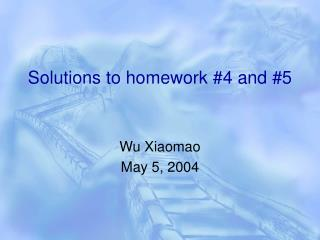# Solutions to homework #4 and #5 - PowerPoint PPT PresentationDownload PresentationSolutions to homework #4 and #5

Solutions to homework #4 and #5Download Presentation## Solutions to homework #4 and #5

- - - - - - - - - - - - - - - - - - - - - - - - - - - E N D - - - - - - - - - - - - - - - - - - - - - - - - - - -
##### Presentation Transcript

1. Solutions to homework #4 and #5 Wu Xiaomao May 5, 2004

2. 12.1. Define the results of performing and for two polyhedral objects in the same way as the result of performing was defined in section 12.2. Explain how the resulting obje-cts is constrained to be a regular set, and sp-ecify how the normal is determined for each of the object’s faces.

3. Solution: • The union operation is defined as: A B=closure(interior(A B)) Nb A B B A Na Nb B A B A Na Nb A B B A Na

4. The union operation is defined as: A B=closure(interior(A B)) Nb A B N A B Na Nb A A B B Na Nb A B A B Na

5. 9.13 Explain why an implementation of Boo-lean set operations on quadtrees or on octr-ees does not need to address the distinction between the ordinary and regularized opera-tions described in Section 12.2

6. Solution: The reason why we need to distinguish between ordinary and regularized Boolen set operations is that we want to resulting objects to be a regularized objects( no dangling edges or dangling surfaces). In quadtrees or octrees representation, objects are expressed in a discrete way. Here no boundary or surface concept is concerned. The Boolean set operation is done by compare the nodes one by one.

7. 12.8 Describe how to perform point classifi-cation in primitive instancing, b-rep, spatial occupancy enumeration, and CSG

8. Solution: • Primitive instancing: • As primitive instance is created by codes, the programmer should design a subroutine for point classification and embed it in the primitive instance. The user can load these subroutines when he/she what to do a point classification.

9. 2. b-rep A B 1 Odd intersection points: In Even intersection points: Out 2 3 4 E C F D

10. 3. Spatial occupancy enumeration Search the quadtree/octree, if point A belongs to one of the empty node, then point A is outside. If point A belongs to one of the full node, continue searching the neighbors of point A (minimum unit). A is on the boundary if one of A’s neighbor is a empty node. A is inside if all its neighbors are full nodes or within a full node.

11. 4. CSG Determine whether the point is inside/outside/ on the boundary for each primitive. Let that point join the Boolean set operation to determine its relation to the object.

12. Supplemental problem: V=16 E=24 F=11 V-E+F=3 V=16 E=28 F=14 V-E+F=2

13. Supplemental problem: V=16 E=24 F=11 H=1 C=1 G=0 V-E+F-H=2 2(C-G)=2 V-E+F-H=2(C-G)